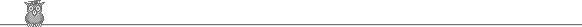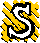### 27th USAMO 1998A1.  The sets {a1, a2, ... , a999} and {b1, b2, ... , b999} together contain all the integers from 1 to 1998. For each i, |ai - bi| = 1 or 6. For example, we might have a1 = 18, a2 = 1, b1 = 17, b2 = 7. Show that ∑1999 |ai - bi| = 9 mod 10.A2.  Two circles are concentric. A chord AC of the outer circle touches the inner circle at Q. P is the midpoint of AQ. A line through A intersects the inner circle at R and S. The perpendicular bisectors of PR and CS meet at T on the line AC. What is the ratio AT/TC?A3.  The reals x1, x2, ... , xn+1 satisfy 0 < xi < π/2 and ∑1n+1 tan(xi - π/4) ≥ n-1. Show that ∏1n+1 tan xi ≥ nn+1.B1.  A 98 x 98 chess board has the squares colored alternately black and white in the usual way. A move consists of selecting a rectangular subset of the squares (with boundary parallel to the sides of the board) and changing their color. What is the smallest number of moves required to make all the squares black?B2.  Show that one can find a finite set of integers of any size such that for any two members the square of their difference divides their product.B3.  What is the largest number of the quadrilaterals formed by four adjacent vertices of an convex n-gon that can have an inscribed circle?To avoid possible copyright problems, I have changed the wording, but not the substance, of the problems.

USAMO home

© John Scholes
jscholes@kalva.demon.co.uk
5 May 2002